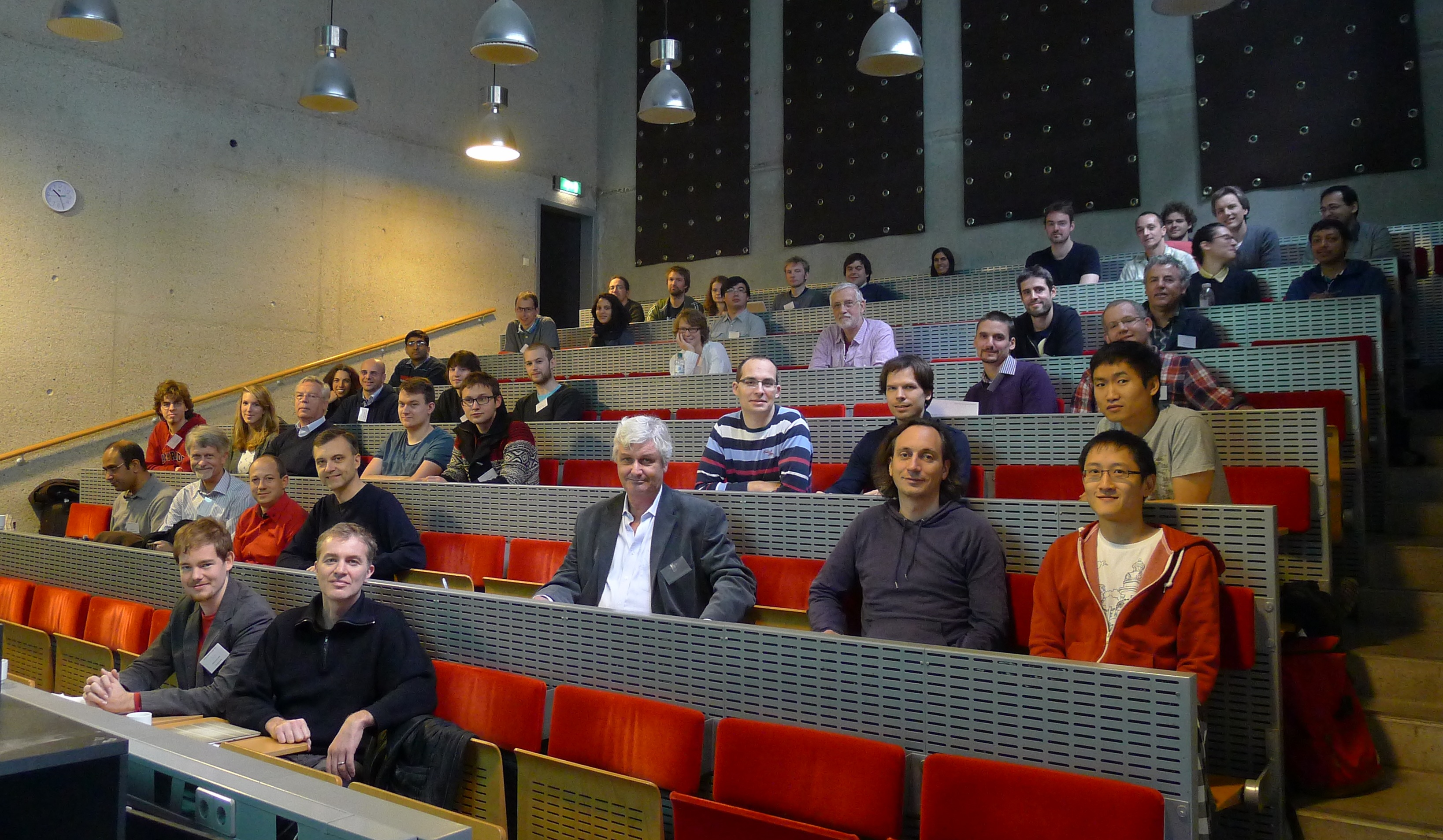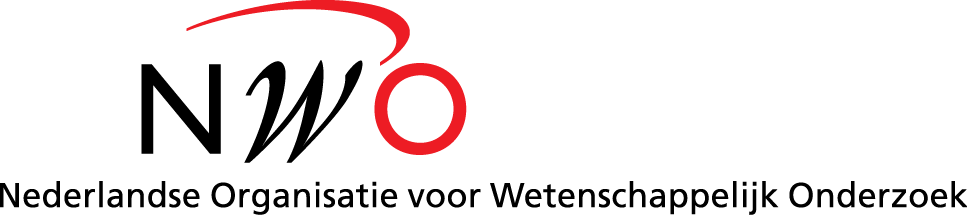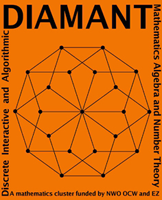# Utrecht Graphs Workshop

The meeting took place at Utrecht University on Thursday, 31 October and Friday, 1 November 2013.### Programme

• Maria Axenovich (Karlsruhe Institute of Technology), "Twins in graphs and sequences".
• Abstract: Two identical disjoint subsequences of a given sequence $$S$$ are called twins of $$S$$. Let $$t(n)$$ be the largest integer $$k$$ such that each binary sequence of length $$n$$ contains twins of length $$k$$ each. In a work with Y. Person and S. Puzynina we show that $$t(n) = n/2 (1-o(1))$$. I will discuss this result, its generalizations for sequences, and twin problem in graphs.

• Aart Blokhuis (TU Eindhoven), "On the $$q$$-analogs of the Kneser graph, their large cocliques and their chromatic number".
• Abstract: One of the famous results in graph coloring is Lovász's proof of the Kneser conjecture concerning the chromatic number of the Kneser graph $$K(n,k)$$ whose vertices are the $$k$$-subsets of an $$n$$-set being adjacent if they are disjoint, in other words 'far away' in the Johnson Scheme. Other famous results are the Erdős-Ko-Rado Theorem, and the Hilton-Milner Theorem that characterize the largest and second largest (maximal) cocliques in this graph.

In this talk I will give a number of recent extensions of these results to $$q$$-analogs of the Kneser graph -- the most studied of these is the graph on the $$k$$-subspaces of an $$n$$-dimensional vector space (over a finite field), being adjacent if their intersection is $$\{0\}$$.

• Hajo Broersma (Universiteit Twente), "On the toughness of graphs".
• Abstract: The toughness of a graph measures the way a graph can fall apart into a number of components by deleting a set of vertices together with the incident edges. We will give the formal definition in the talk. Since its introduction in a paper due to Chvátal in 1973 many results have been obtained that relate the toughness of a graph to its cycle structure, but many open problems remain. In the talk we will discuss old and new results involving the toughness of graphs and we will discuss the progress on the main open conjectures.

• Louis Esperet (CNRS, G-SCOP), "Covering cubic bridgeless graphs with perfect matchings".
• Abstract: A conjecture of Berge and Fulkerson (1971) states that every cubic bridgeless graph contains 6 perfect matchings covering each edge precisely twice, which implies that every cubic bridgeless graph has 3 perfect matchings with empty intersection (this weaker statement was conjectured by Fan and Raspaud in 1994). I will show that if 4/5 of the edges of every cubic bridgeless graph can be covered by 3 perfect matchings, then Fan-Raspaud conjecture holds, confirming a recent conjecture of W. Tang, C.Q. Zhang, and Q. Zhu. I will also prove that for any $$2\le t \le 4$$ and for any real $$\tau$$ lying in some appropriate interval, deciding whether a fraction of more than (resp. at least) $$\tau$$ of the edges of a given cubic bridgeless graph can be covered by $$t$$ perfect matching is an NP-complete problem. This proves another conjecture of W. Tang, C.Q. Zhang, and Q. Zhu.

I will also mention a construction of an infinite family of snarks whose edge-set cannot be covered by 4 perfect matchings (only two such graphs where known beside the Petersen graph). This construction is also interesting in the study of the shortest cycle cover of snarks.

Joint work with G. Mazzuoccolo.

• Tom Kaiser (University of West Bohemia), "Colouring quadrangulations of projective spaces".
• Abstract: Youngs proved in 1996 that any quadrangulation of the projective plane is either bipartite, or 4-chromatic. We extend the definition of a quadrangulation to higher dimensions, and prove that any graph $$G$$ which embeds as a quadrangulation in the real projective space $$P^n$$ has chromatic number $$n+2$$ or higher, unless $$G$$ is bipartite. The family of quadrangulations of projective spaces includes all complete graphs and all Mycielski graphs. We obtain a new proof of the Lovász-Kneser theorem by showing that certain projective quadrangulations are homomorphic to the Schrijver graphs. Finally, we show that (in contrast to the two-dimensional case) the chromatic number of quadrangulations of $$P^n$$ is not bounded by any function of $$n$$. The talk is based on joint work with Matěj Stehlík.

• Dan Král' (University of Warwick), "FO limits of trees".
• Abstract: Nešetřil and Ossona de Mendez introduced a new notion of convergence of graphs called FO convergence. This notion can be viewed as a unification of notions of convergence for dense and sparse graphs. In particular, every FO convergent sequence of graphs is convergent in the sense of left convergence for dense graphs, and every FO convergent sequence of graphs with bounded maximum degree is convergent in the Benjamini-Schramm sense. FO convergent sequences of graphs can be associated with a limit object called modeling. Nesetril and Ossona de Mendez showed that every FO convergent sequence of trees with bounded depth has a modeling. We extend this result to all FO convergent sequences of trees and discuss possibilities for further extensions.

The talk is based on a joint work with Martin Kupec and Vojtech Tuma.

• Alex Scott (University of Oxford), "Hypergraphs of bounded disjointness".
• Abstract: A $$k$$-uniform hypergraph is said to be intersecting if no pair of edges is disjoint. The maximal size of an intersecting $$k$$-uniform hypergraph with a given groundset is given by the beautiful and well-known theorem of Erdős, Ko and Rado.

A $$k$$-uniform hypergraph is $$s$$-almost intersecting if every edge is disjoint from exactly $$s$$ other edges. Gerbner, Lemons, Palmer, Patkós and Szécsi made a conjecture on the maximal number of edges in such a hypergraph. We prove a strengthened version of this conjecture and determine the extremal graphs. We also give some related results and conjectures.

Joint work with Elizabeth Wilmer.

• Benny Sudakov (ETH and UCLA), "Counting and packing Hamilton cycles".
• Abstract: We present a general method, based on estimates for permanents of matrices, for counting and packing Hamilton cycles in dense graphs and oriented graphs. We utilize this approach to prove several new extremal results, and also to derive new and conceptually simple(r) proofs of some known results in this area.

Joint with A. Ferber and M. Krivelevich

• Carsten Thomassen (Technical University of Denmark), "The weak 3-flow conjecture and some applications".
• Abstract: Tutte's 3-flow conjecture says that every 4-edge-connected graph has an orientation such that, for each vertex $$x$$, the indegree of $$x$$ equals the outdegree of $$x$$ modulo 3. In 1988 Jaeger suggested a weaker conjecture obtained by replacing 4 by a larger (universal) number and called that the weak 3-flow conjecture. He also suggested a stronger conjecture, called the circular flow conjecture.

In this talk we indicate a proof of the weak circular flow conjecture (and hence also the weak 3-flow conjecture) and discuss its applications to graph decomposition, group flow, and factors modulo $$k$$.

The programme also included contributed short talks by Nieke Aerts, Kunal Dutta, Ararat Harutyunyan, Tony Huynh, Domenico Labbate, Jesper Nederlof, Viresh Patel and Guus Regts.

The detailed schedule and abstracts are found in the workshop booklet. The short programme.

#### Registered participants

The organisers and invited speakers, plus Aida Abiad (Tilburg), Marien Abreu (Potenza), Nieke Aerts (TU Berlin), Nikhil Bansal (TU Eindhoven), Evan DeCorte (TU Delft), Josse van Dobben de Bruyn (Leiden), Kunal Dutta (MPI Saarbrücken), Roberto Fernández (Utrecht), Murat Firat (VU Amsterdam), Dion Gijswijt (TU Delft), Marinus Gottschau (TU München), Grzegorz Gutowski (Jagiellonian), Willem Haemers (Tilburg), Ararat Harutyunyan (Oxford), Tony Huynh (Sapienza), Leo van Iersel (CWI), Lotte de Jonker (Amsterdam), Judith Keijsper (TU Eindhoven), Steven Kelk (Maastricht), Domenico Labbate (Potenza), Jan van Leeuwen (Utrecht), Nela Lekic (Maastricht), Anshui Li (Utrecht), Killian Matzke (TU München), Jesper Nederlof (Utrecht), Neil Olver (VU Amsterdam), Viresh Patel (Birmingham), Murray Patterson (CWI), Christos Pelekis (TU Delft), Helena Peña (Greifswald), Rudi Pendavingh (TU Eindhoven), Teresa Piovesan (CWI), Jorn van der Pol (TU Eindhoven), Guus Regts (CWI), Mayank Singhal (CAU Kiel), Cristian Spitoni (Utrecht), Matěj Stehlík (Grenoble), Leen Stougie (VU Amsterdam), Siamak Taati (Utrecht), Christoph Temmel (VU Amsterdam), Antonios Varvitsiotis (CWI), Kees de Vreugd.